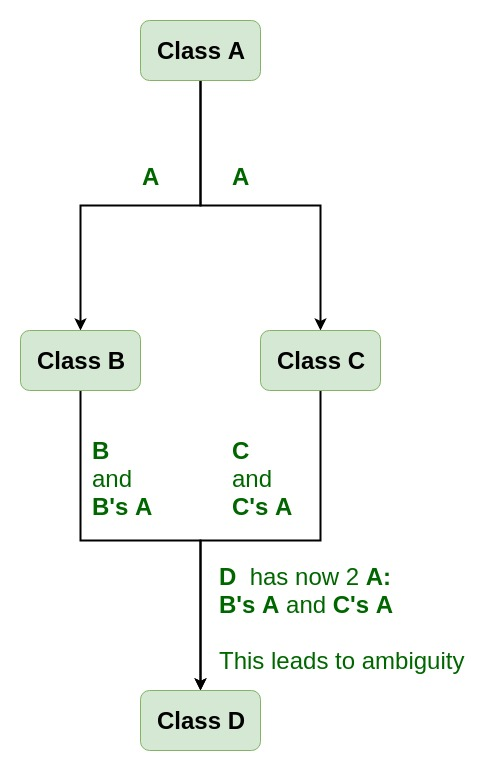# Virtual base class in C++

• Difficulty Level : Medium
• Last Updated : 14 Mar, 2019

Virtual base classes are used in virtual inheritance in a way of preventing multiple “instances” of a given class appearing in an inheritance hierarchy when using multiple inheritances.

Need for Virtual Base Classes:
Consider the situation where we have one class A .This class is A is inherited by two other classes B and C. Both these class are inherited into another in a new class D as shown in figure below.As we can see from the figure that data members/function of class A are inherited twice to class D. One through class B and second through class C. When any data / function member of class A is accessed by an object of class D, ambiguity arises as to which data/function member would be called? One inherited through B or the other inherited through C. This confuses compiler and it displays error.

Example: To show the need of Virtual Base Class in C++

 `#include ``using` `namespace` `std;`` ` `class` `A {``public``:``    ``void` `show()``    ``{``        ``cout << ``"Hello form A \n"``;``    ``}``};`` ` `class` `B : ``public` `A {``};`` ` `class` `C : ``public` `A {``};`` ` `class` `D : ``public` `B, ``public` `C {``};`` ` `int` `main()``{``    ``D object;``    ``object.show();``}`

Compile Errors:

```prog.cpp: In function 'int main()':
prog.cpp:29:9: error: request for member 'show' is ambiguous
object.show();
^
prog.cpp:8:8: note: candidates are: void A::show()
void show()
^
prog.cpp:8:8: note:                 void A::show()
```

How to resolve this issue?
To resolve this ambiguity when class A is inherited in both class B and class C, it is declared as virtual base class by placing a keyword virtual as :

Syntax for Virtual Base Classes:

```Syntax 1:
class B : virtual public A
{
};

Syntax 2:
class C : public virtual A
{
};
```

Note: virtual can be written before or after the public. Now only one copy of data/function member will be copied to class C and class B and class A becomes the virtual base class.
Virtual base classes offer a way to save space and avoid ambiguities in class hierarchies that use multiple inheritances. When a base class is specified as a virtual base, it can act as an indirect base more than once without duplication of its data members. A single copy of its data members is shared by all the base classes that use virtual base.

Example 1

 `#include ``using` `namespace` `std;`` ` `class` `A {``public``:``    ``int` `a;``    ``A() ``// constructor``    ``{``        ``a = 10;``    ``}``};`` ` `class` `B : ``public` `virtual` `A {``};`` ` `class` `C : ``public` `virtual` `A {``};`` ` `class` `D : ``public` `B, ``public` `C {``};`` ` `int` `main()``{``    ``D object; ``// object creation of class d``    ``cout << ``"a = "` `<< object.a << endl;`` ` `    ``return` `0;``}`

Output:

```a = 10
```

Explanation :The class A has just one data member a which is public. This class is virtually inherited in class B and class C. Now class B and class C becomes virtual base class and no duplication of data member a is done.

Example 2:

 `#include ``using` `namespace` `std;`` ` `class` `A {``public``:``    ``void` `show()``    ``{``        ``cout << ``"Hello from A \n"``;``    ``}``};`` ` `class` `B : ``public` `virtual` `A {``};`` ` `class` `C : ``public` `virtual` `A {``};`` ` `class` `D : ``public` `B, ``public` `C {``};`` ` `int` `main()``{``    ``D object;``    ``object.show();``}`

Output:

```Hello from A
```

My Personal Notes arrow_drop_up